﻿ Inter-satellites Optical Communication Systems for DefensePublications are Open
Access in this journal
Article Versions
Export Article
• Normal Style
• MLA Style
• APA Style
• Chicago Style
Research Article
Open Access Peer-reviewed

### Inter-satellites Optical Communication Systems for Defense

João Miguel Trindade, João Paulo N. Torres , António Baptista, Mª João Martins
American Journal of Electrical and Electronic Engineering. 2017, 5(3), 108-119. DOI: 10.12691/ajeee-5-3-6
Published online: July 01, 2017

### Abstract

Satellite communication systems are increasingly more used by the society in many applications, and its development is a high priority for the scientific community. The need for increasing bandwidth to satisfy the users’ needs, makes inter-satellites Free Space Optics (FSO) communication preferred instead of traditional radio frequency links. The inter-satellites communication links with high bit rates and low power is a determining factor in the performance of these systems. As such, communications occur over long distances and high altitude orbits, which requires optical sources with highly collimated and coherent beams in order to ensure a better link between the transmitter and the receiver with low emission power. This paper involves the development of two experimental blocks for implementing an inter-satellite optical communication system using a semiconductor laser type. It aims at the definition and analysis of the elements of the two circuits, at the transmitter subsystem and includes the design for the production of printed circuit boards (PCB). Moreover, experimental tests were performed to validate the results obtained in the simulations.

### 1. Introduction

The demand for diversified services, such as the use of high speed internet services, videoconference and watching programs or videos directly on the Internet (streaming) has resulted in a dramatic increase of the bandwidth required, as the data rate transmission is determined by the available bandwidth 1, 2.

Satellites have nowadays several applications of great importance to the society, such as in telecommunications, in weather forecast and in earth’s surface observation, on GPS coordinates navigation (Global Positioning System), and even in space research. On the military scope, the mentioned applications referred above are relevant in recognition and surveillance in the theatre of operations (TO). The information collected in real time may represent and advantage to the military leadership enabling their correct decision process 2.

The wide spectrum of optical communications (without regulation so far), unlike the RF communications, represents an additional advantage due to the possibility of data rate transmission of the order of dozens of Gbps 1.

A crucial device to establish the communication between the satellites is the optical source. Semiconductor lasers are often considered ideal sources of transmission due to their high efficiency in the conversion of electrical into optical power, their small size, their easy integration and their low energy consumption. Their low cost is also a reason to select them as the optical source to be used 3, 4.

The purpose of this paper is to define a transmitter subsystem, operating at optical frequencies, that may be integrated in an optical satellite intercommunication system, simulate the transmitter subsystem, and build and test the several blocks that constitute this subsystem.

### 2. State of Art of the Optical Communications Inter-satellites

2.1. Satellite Systems Evolution

The development of lasers since the 60’s dcade of the XXth century, triggered the interest of international agencies on this technology. The European Space Agency (ESA) developed the first studies for the use of lasers in inter-satellite communications. In the beginning of 1991 the first unidirectional inter-satellite communication link was established through the program SILEX. The connection was established between the Pastel optical terminals, on board of the French ground observation satellite SPOT-4 and OPALE, installed in the European communications satellite ARTEMIS 5, 6. The satellites SPOT-4 and ARTEMIS were set in a low earth orbit (LEO) and in a geostationary orbit, respectively. The distance between them was approximately 45000 km and while SPOT-4 transmitted images at 50 Mbps rate to ARTEMIS, this satellite would retransmit them to the Earth almost in real time. To establish this connection a laser with a power transmission of 60 mW was used, with a wavelength of 0.8 µm and OOK modulation 4, 7.

In 2008 a successful demonstration of an optical inter-satellite connection (between satellites TerraSAR-X and NFIRE) on the low earth orbit was established. The LCTs enabled the increase of the users’ data traffic compared to the radiofrequency connections and they are, nowadays, the most used modules in the industry to establish inter-satellite optical communications 4, 8.

2.2. Conditioning Factors to the Inter-satellites Communication

The satellites can be classified according to their purpose and orbit type.

Regarding the purpose, there are communication satellites which are responsible for voice, TV and Internet transmission. There are also meteorological, scientific, navigation, earth observation and military satellites. Military satellites are essential in navigation assistance and in the positioning of military forces as well as in surveillance and recognition tasks, contributing to the enemy’s surprise effect 9.

The type of orbit depends the on their distance to the Earth. In Figure 1 the three types of orbits are presented.

• Figure 1. Three types of satellite orbits

• Low Earth Orbit (LEO) satellites correspond to a distance of 180 to 2000 km from the earth’s surface. This type of satellites is ideal for military observations, for meteorological data collection and for communications. They consume less energy and they have lower launching costs.

• Medium Earth Orbit (MEO) satellites correspond to 2000 km to 25800 km above the earth’s surface. These are generally navigation (GPS), communication and scientific satellites. Their coverage area is wider than LEO’s.

• Geostationary Earth Orbit (GEO) satellites: they orbit the Earth at a 35800 km distance and their orbital period is 24 hours 10, 11, synchronous with the Earth rotation period They cover a wider area, but are big expensive devices, which demand a lot of energy to be launched into space.

In general, the orbits at a higher altitude are more stable due to less interference caused by atmospheric density and by gravitational fluctuations. The gravitational fields of the celestial bodies (such as the Moon and the Sun), the solar radiations and the centrifugal force effect generated by the Earth’s rotation movement can affect the orbital stability of the satellites 12.

2.3. Basic Parameters of the Inter-satellites Communication Systems

The optical communications established between satellites occur mainly in the exosphere atmospheric layer (above 500 km) and although the temperatures reach as much as 1000°C, there is no danger of overheating for the satellites, as the atmosphere is extremely rarefied causing a very low heat exchange. This way there is no attenuation caused by the atmosphere and so, the electromagnetic field generated by the transmitter propagates almost in vacuum.

In an optical communication, system the power of the optical signal transmitted is affected by many factors before reaching the receiver. These factors include loss of connection route in t free space (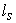), pointing losses (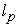) and background radiation. The pointing losses are a result of the difference between the beam direction and the satellite position. Regarding the losses due to the divergence of the beam, they can be calculated considering the opening angles (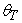and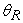) and the diameter of the antenna (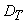and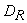) 5, 13.

The power flow equation describes the performance of this system. The power of the signal received (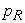) [W] depends on the power transmitted (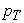) [W], on the transmitting antenna gain (), on the receiving antenna gain (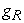), on the route losses in the free space and on the transmitter (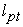) and the receiver (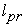) pointing losses.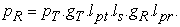(1)

The antenna gain (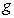) depends on the lens optical efficiency (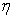) and the antenna’s opening diameter (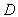) [m] as given by: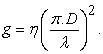(2)

This way, the transmitting (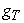) and receiving () gain is calculated considering the opening diameter of the transmitting and receiving antennas, respectivelyand. The opening angles can be calculated through: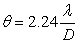(3)

whereandare the opening angles of the transmitting and receiving antenna in radians. The pointing losses are given by: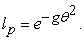(4)

The route losses in free space () depend on the length of the link (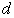) [m] and are given by: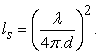(5)

In general, considering the analysis of the above expressions, the advantages of the FSO system are a result of the basic characteristics of the laser beam. As can be inferred from (2) and (5), for higher frequencies higher transmitting and receiving gains are obtained and less free space losses occur 5, 13.

### 3. Characteristics of the Transmitting Subsystem

3.1. Blocks Description and Diagram

The current FSO systems operate typically in wavelengths in the near infrared spectrum, between 800 and 1600 nm. There are some special windows of wavelengths of operation, more specifically 850, 1310 and 1550 nm. The 1310 and 1550 windows match the standard transmitting windows of the optical fiber communication systems, so the majority of the FSO commercial systems operate in these two windows in order to use the most common components. The Ultra-Violet (UV) bandwidth was recently considered for the FSO systems, because apart from being less subject to pointing errors and to beam interference it has the advantage of being less sensible to interferences caused by background radiation 14, 15. Figure 2 shows the electromagnetic spectrum where we can observe the ultraviolet, visible and infrared areas.

• Figure 2. Electromagnetic Spectrum

The transmitting system is composed by the optical source, the modulator and the optical amplifier, leading to the optical beam at the output, as we can see in Figure 3. The channel encoding can be performed optionally before modulation; this way the bits generated by the source are first codified and later modulated. In order to increase the optical intensity of the laser beam, which was previously modulated, an optical amplifier can be used. Then, the light beam is transmitted by an optical antenna.

• Figure 3. Block diagram of the optical transmitter

The optical source used in FSO systems is generally a semiconductor laser, although some manufacturers use the high power LED technology with beam collimators for that purpose. The most used semiconductors laser types are: the FP (Fabry-Perot), the DFB (Distributed Feedback) and the VCSEL (Vertical Cavity Surface Emitting Lasers).

3.2. Historical evolution of the semiconductor lasers

In 1960 Maiman demonstrated the performance of the first laser using a ruby crystal, operating in the visible. The first semiconductor laser, an homogeneous structure device, which used a p-n junction of GaAs, was developed in 1962 by Hall 4, 16, 17.

A perpendicular p-n junction plan with two polished end surfaces has turned the semiconductor into a small FP cavity. However, the fact that the FP lasers need a high threshold current caused heating problems in the system 18.

In 1969, the first solution to operate at room temperature was devised by using hetero structure semiconductor lasers, type GaAs/AlGaAs..

In 1990, the need to develop laser amplifiers arose, in order to establish long haul communications using optical fibers. The need to transmit several signals simultaneously in the same physical space – Wavelength Division Multiplexing (WDM) – led to the development of DFB lasers and DBR (Distributed Bragg Reflector) lasers. This kind of lasers, with single mode operation increased the frequency stability of these systems 4, 19.

Nowadays the most common laser type is the semiconductor, which has wide application. These type of lasers is usually preferred to other types, due to their great efficiency of converting electrical into optical power, their small size, light weight and possibility of direct modulation types of semiconductor lasers.

In telecommunication, systems the FP laser and the DFB laser are the most commonly used light sources. However, for systems in which data transmission is predominant and when costs are concerned, such as the GbE (Gigabit Ethernet), the FP laser and the VCSEL are the most used 21. In the next section a description of the composition and main characteristics of the most used types of semiconductor lasers in optical connections such as the FP, the DFB and the VCSEL is provided. Laser FP (Fabry-Perot)

The simplest structure of a semiconductor laser is an FP laser, as represented in Figure 4.

• Figure 4. Structure of an FP laser

To help confining the bearers to the active region (GaAs), the surrounding doped areas p and n, which in this case are made of AlGaAs, have a large forbidden band. In the edges two surfaces mirrors are used to control the light output.

The FP lasers are not composed by frequency selective elements, which mean they are not Multiple-Longitudinal Mode lasers (MLM). However, by reducing the size of the cavity it is possible to get a behavior close to a single mode laser 4, 21.

3.2.1. DBF (Distributed Feedback) Laser

This laser has an active area similar to that of a FP laser, with a built-in corrugation area that acts like a mode filter. Instead of concentrating the reflexivity in the edges of the cavity, its reflection properties are distributed along the active area, that is, along the length of the cavity, as presented in Figure 5.

• Figure 5. Structure of a DFB laser

The reflections required for laser operation are due to the grating, which enables only a specific wavelength. Therefore, the DBF lasers are Single Longitudinal Mode (SLM) lasers. These devices are coated with antireflective materials on the front side and with a high reflexivity coating on the rear side, in order to guarantee that the light comes out only in one direction.

3.2.2. VCSEL Laser

The VCSEL laser is a single laser mode and is geometrically different from the previous ones. The first major difference, as we can see in Figure 6, is that the laser transmits perpendicularly to the surface of the structure.

• Figure 6. VCSEL laser structure

The active area is placed between two surfaces of high reflectivity, DBR “mirrors”. This high reflectivity is obtained through the formation of layers with overlapping of multiple materials with different refraction indexes. 3, 22.

Table 1 presents some characteristics of the three types of lasers.

3.3. Modulation Techniques

The modulator converts the data to transmit into a new established standardized format. Its main purpose is to obtain the maximum amount of data in the narrower bandwidth possible. There are different types of modulation schemes adequate to the FSO communication systems, such as OOK (On-Off Keying), PPM (Pulse Position Modulation) and PSK (Phase-Shift Keying) 1.

3.3.1. PSK Modulation

PSK modulation is predominant in the most recent inter-satellite optical connections and relies on the phase shifts of the modulated signal for the transmission of the different bits. The modulation techniques resulting from the PSK technique are BPSK (Binary Phase-Shift Keying), DPSK (Differential Phase-Shift Keying) and QPSK (Quadrature Phase-Shift Keying).

In BPSK modulation each phase shift of 180 degrees corresponds to a transition of the NRZ (Non-Return-to- Zero) signal from “0” to “1” (or vice-versa, according to the previous phase).

In DPSK modulation the phase shift only occurs when a “0” bit is sent. Thus, each “0” bit sent, usually corresponds to a phase shift of 180°. The BPSK and DPSK techniques are shown in Figure 7.

• Figure 7. PSK modulation techniques: (a) NRZ signal, (b) BPSK signal, (c) DPSK signal

In QPSK modulation it is possible to transmit bits by symbol, because phase and quadrature parameters are used in the modulated wave, making this implementation technique more complex than the previous ones.

3.3.2. OOK Modulation

OOK modulation is a special case of amplitude modulation. from, It is a binary technique in which each time slot -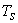– corresponds to a bit. The presence of a laser impulse indicates the emission of a bit “1”, while the “0” bit is indicated by a null impulse (absence of signal), as represented in Figure 8.

• Figure 8. OOK signal for NRZ impulses

3.3.3. PPM Modulation

M-PPM modulation consists in a time division of the transmission of a symbol in equal M time slots, where M is the modulation sequence. In order to represent a certain symbol, a pulse is sent in one of those M time slots, as illustrated in Figure 9.

• Figure 9. M-PPM signal

### 4. Circuits Simulation and Testing

Two types of circuits are used to transmit the information using the laser source. The first circuit, with discrete components, operated below 10 MHz and the second circuit, composed by integrated components, was designed to reach frequencies in the GHz band.

4.1. Circuit with Discrete Components

The first circuit consists of three stages: the first stage is a differential pair, the second by two inverter stages and the third by the laser.

4.1.1. Differential Pair

The differential pair which can be observed in Figure 10 is a consists of two bipolar junction transistors 2n3904 (Q1 and Q2). Their purpose is to work as a differential amplifier, as both transistors are in the operation area of direct active zone.

• Figure 10. Differential pair with bipolar transistors

In Figure 11 we can see a simpler version of Figure 10 to simplify the DC analysis of the differential pair.

• Figure 11. Differential pair parameters

It is possible to observe all the parameters that constitute the differential pair in Figure 11. These parameters are highly important for the theoretical calculation, enabling the interpretation of the differential pair operation. Knowing that the sum of the power failures in a closed circuit is zero as stated in Kirchhoff Voltage Law (KVL), we can get the following expressions: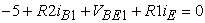(6)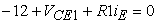(7)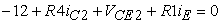(8)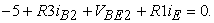(9)

The sum of the currents from the TBJ Q1 and TBJ Q2 transmitters equals the current that crosses R1, that is: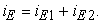(10)

Also knowing that according to the TBJ’s characteristics: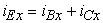(11)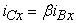(12)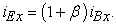(13)

Admitting that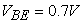and that the current gain figure is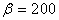for both TJBs the differential pair parameters are obtained.

Table 2 shows the theoretical values obtained and the values simulated in the PSpice program.

The incremental model is valid for small variations of the input signal and when the differential pair works as a linear amplifier. In the differential mode two different voltages are applied at the base of the transistors Q1 and Q2, respectively,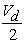and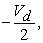whereas in the common mode the voltages applied at the base,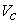are equal in both transistors.

The expressions for the differential and of the common mode gain are given respectively, by: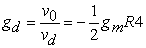(14)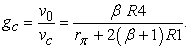(15)

For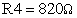we can obtain a differential mode gain of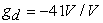in linear units and of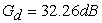in logarithmic units (in module). In (15) with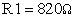we have a common mode gain of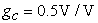in linear units and of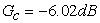in logarithmic units.

The ratio between the differential mode gain and the common mode gain is the Common Mode Rejection Ratio (CMRR), given by the expression: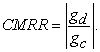(16)

The capacity of the differential amplifier to reject equal signals applied to the inputs Vin+ and Vin- (CMMR) amounts to 38.28 dB.

The TJBs used, are npn type and depending on the polarization condition (direct or inverse) of each junction, they can operate in four different areas: Cut-off area, Direct or Inverse Active area and Saturation area. The collector, base and transmitter currents are equal and positive for both transistors and it was assumed that both transmitter base junctions were conducting and that the voltages at their terminals were equal to 0,7 V. The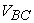voltages in the collector base junctions of the two transistors, although of different value, are both negative. In these conditions, the operation area for both transistors is the direct active area.

To check the circuit’s behavior the PSpice simulator was used, introducing in the circuit input a rectangular impulse ranging between 0 V and 5 V, for frequencies of 0.1 MHz and 10 MHz. In Figure. 12 we can observe the 10MHz pulse.

• Figure 12. Input signal Vin, 10 MHz

The signal at the output of the TBJ Q2 (Vout) collector for the frequency of 0.1 MHz is represented in Figure13.

• Figure 13. Transitional regime of TJB Q2 collector, f=0.1 MHz

For this frequency, the signal presented in Figure 14 was obtained experimentally.

• Figure 14. Tests to the TJB Q2 collector, 0.1 MHz

Comparing the signal obtained in the experimental test with the PSpice simulation signal, we conclude that there is a slight decrease of amplitude of 0.4 V. There was also a change of the duty cycle from 50% to 75 %.

4.1.2. Inverter Stages

The second part of the electrical circuit is composed by two inverter stages. Figure15 shows the two inverter-stages in a simplified version.

• Figure 15. Inverter stages

The two-inverter stages, which are part of the circuit, have different roles. In both stages the inverters are connected in parallel and while the first stage, composed by 3 inverters, has the purpose of stabilizing the input signal sent from the differential pair, the second stage, composed by 15 inverters has the purpose of supplying more current for the laser.

The signal at the output of the first inverter stage for the frequency of 0.1 MHz is represented in Figure16.

• Figure 16. Transitional regime after the 1st inverter stage, 0.1 MHz

Comparing Figure 12 with Figure 16 the signal inversion is confirmed, however the main reason for that stage is the stabilization of the signal.

The signal at the output of the second inverter stage for 0.1 MHz frequency is represented in Figure 17.

• Figure 17. Transitional regime after the 2nd inverter stage, 0.1 MHz

As presented in Figure. 17, the transitional regime of this signal is different, presenting in two time slots two different levels of voltage. The changing behavior of voltage levels is due to the voltage limits V1L and V1H, characteristic of the 7404 inverter. Once the V1L maximum is 0.8 V and the V1H minimum is 2 V, those axis were drawn in Figure 18 chart in order to analyze the observed behavior.

• Figure 18. Characteristics of the 7404 inverter, 0.1 MHz

V1L and V1H of the inverter 7404 are represented in blue in the Figure 18 the curve in green represents the pulse before the 2nd stage of inverters and the one in red represents the pulse after it. Every green signal below the V1L line is considered a logical value “0”, above V1H line the logical value considered is “1” and between those lines, there is a non-defined area.

In the experimental test as the inverters used were the 74HCT04 with different voltage levels, no problem occurred as we can see in Figure 19.

• Figure 19. Results obtained in the test to the output of the 2nd stage inverters, 0.1 MHz

The signal introduced through a signal generator in the experimental circuit is represented in blue, whereas the the signal at the output of the second inverter stage is presented in red.. The red signal is the input signal to the laser.

4.2. Circuit with Integrated Components

Another way of transmitting information using a laser is by exciting it with integrated circuits of advanced technology, projected specifically for that purpose. The integrated circuits MAX3643 and DS1865 are, respectively, a circuit that controls the laser excitation current and a circuit that checks the light power transmitted by the laser and adjusts the drive current in order to keep the light power constant. Figure20 presents the circuit, which enables the information transmission with those integrated circuits, in this case for high frequencies.

• Figure 20. Circuit for high frequencies

In Figure 20 there are several components that involve the integrated circuit MAX3643. These components (resistors, capacitors and inductors) determine the values of currents, voltages and frequencies in the circuit’s operation.

Resistors R1 and R2 constitute a voltage splitter of the input signal (Vin) protecting both the integrated circuit and the laser. The signal transmitted by that voltage splitter is the input signal in the integrated circuit MAX3643, more specifically in ports IN+ and BEN+.

Port IN+ is responsible for the acceptance of information bits, while port BEN+ (when active) is responsible for the emission of light through the laser. In this case, IN+ and BEN+ ports were connected to the same circuit point, which means that when the Vin input signal is in low level, the laser is off. When the Vin input is high, the BEN+ input enables the laser excitation according to the dimensioning performed at ports MODSET and BCMON, as we can see ahead.

Once the input maximum voltage in the integrated circuit MAX3643 (in pin IN+) is 0.8 V, from (17) one obtains: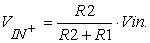(17)

For the input signal Vin, the same rectangular pulse was considered, but for calculation effects only its maximum value, 5 V was taken into account. For a ratio between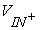and Vin of 0.16, the resistors values used, were 100 Ω for R2 and 560 Ω for R1.

Taking into account that the laser has a current threshold value varying between 24 mA and 30 mA and an operating current between 33 mA and 40 mA, the maximum current at the output of the integrated MAX3643, on pin IMAX (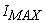) is given by (18).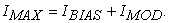(18)

The current modulation,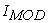, and the forward bias current,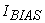, are turned off if their sum exceeds the limit set by the resistor of the pin IMAX (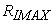). The value of 33 mA was chosen for currentand for the currentthe value of 5 mA. It is now possible to control the maximum current to the output of the controller by choosing appropriately the value of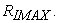For a currentof 38 mA the use of a resistor in the pin IMAX 15 kΩ, will prevent the laser from burning.

To be able to control both the chain of modulationas the forward bias current, two resistors are introduced in the circuit, respectively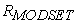and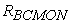. The resistor value ofcan be obtained, according to the specifications of the controller MAX3643, through (19).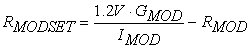(19)

where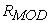and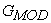, are typically 50 Ω and 88 mA/mA and denote respectively, the internal resistor of the pin MODSET and current gain modulation. The resistor value ofshould be chosen to produce the maximum current of modulation for the operating temperature of the laser. For a chain of modulationof 5 mA, the resistor valueis 21.07 kΩ. Thus, it is assumed for the resistorthe value of 18 kΩ, which corresponds to a chain of modulationof 5.85 mA.

The resistoris obtained from the current gain of polarization,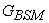, and stating that the voltage at the terminals,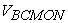, must be less than 1.4 V. The resistoris determined according to the specifications of the controller MAX3643, through (20).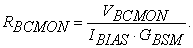(20)

For a currentof 33 mA, with a gainof 17 mA/A, a value of 3.03 kΩ for resistoris obtained. A value of 3.3 kΩ for, leads to a currentof 30.3 mA, higher than the stream of threshold (30 mA).

The pin OUT+ and the pin BIAS+ are the pins for the currents of modulation and polarization respectively. Damping resistors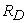(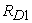to the pin OUT+ and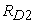for the pin BIAS+) connected to these pins, in series, control the current through the laser. The sum of the value of this resistor with the equivalent resistor of the laser,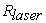, should be approximately 15 Ω. Since the typical resistance of a laser FP varies between 4 Ω to 6 Ω, a resistorandof 10 Ω must be used.

The pins OUT- and BIAS- allow the output of the chain of modulation and the forward bias current respectively, when the pin entry BEN is at the low level. These variations of current, lead to the pin OUT- being connected to a resistor of 15 Ω and a switching diode (1N4148) the node of the laser and the pin BIAS- linked to a resistor of 10 Ω, a diode 1N4148 and a capacitor (C1) of 10 pF VCC (3.3 V), preventing the current to flow in that direction.

In the case of operation at high frequencies, a link RC (RCOMP and CCOMP) connected in parallel between the cathode of the laser and the ground should be introduced to reduce any possible distortion in the duty-cycle of the laser, caused by its parasitic inductances. For the value of 75 Ω was used for RCOMP and the value of 27 pF for CCOMP, resulting in a cut-off frequency of 7.86 MHz.

To transmit data rates above 1 Gbps, it is recommended to use a link RL (RP and LP) connected in parallel between the anode of the laser and VCC. This link leads to an improvement in the response of the times of ascent and descent of the laser and reduces the its instability. The values of RP and LP can be adjusted taking into account the type of laser, however, for experimental studies in the order of GHz the values of 15 Ω and 10 nH, will be used for RP and LP respectively.

### 5. Layout of Circuits

The two circuits considered in this paper were designed in the EAGLE program, version 7.6.0, with the aim of producing Printed Circuit Board (PCB

In Figure 21 the final design for the PCB of the circuit using discrete components is shown.

• Figure 21. PCB project using discrete components

As can be seen in Figure 21, the PCB is composed of two layers; the top layer is represented by red lines and the bottom layer, by the blue lines. In a PCB design, there are considerations to take into account, in particular the width of the lines which, as can be seen in Figure 21, differ on connections made to the components represented by VIN, FICHAS (connectors) and LASER.

The element represented by VIN is a male BNC connector and allows the input of the signal to be fed to the laser. The device represented by "FICHAS", has three connectors for the input signals: in port 1 signal ground (GND), in port 2 a DC voltage of 5 V (VCC) and at port 3 a voltage of 12 V (12VCC). These two components have connecting lines with larger width to decrease resistance. Since the laser is a key part of this circuit, its connection lines are also wider, allowing faster responses.

In Figure 22 is represented the final design for the PCB of the second circuit with integrated components responsible for the set-up and control of the laser.

• Figure 22. Second circuit PCB project

As can be seen in Figure 22, there are only two types of signals that connect to the component FICHAS: at port 1 a DC voltage of 3.3 V that powers the integrated MAX3643 and at port 2 signal ground (GND).

### 6. Conclusions

This paper aims at describing the optical transmitter subsystem of an optical intersatellite link. Two PCBs are presented for the low frequency simulation. Experimental tests were performed and compared to the simulation results.

It was found that the FP lasers, despite having a simple structure, can simulate the behavior of a single mode laser when the size of its cavity decreases. Thus, a laser FP of complex structure, AlGaInP, was used to establish communication with both circuits.

In conclusion, the results obtained were satisfactory. The main problems were the differences in the parameters of the components of the PSpice simulator and experimental results, which presented some differences.

A circuit with integrated components was designed.

### Acknowledgments

This work was supported by national founds through the Fundação para a Ciência e a Tecnologia (FCT) of the Portuguese Government with reference UID/EEA/50008/2013.

### ReferencesThis work is licensed under a Creative Commons Attribution 4.0 International License. To view a copy of this license, visit http://creativecommons.org/licenses/by/4.0/